Скачать презентацию Cyclographic and stereographic projection What is important

4-stereonet-projection_-_copie.ppt

• Размер: 4.7 Mегабайта
• Количество слайдов: 43

## Описание презентации Cyclographic and stereographic projection What is important по слайдам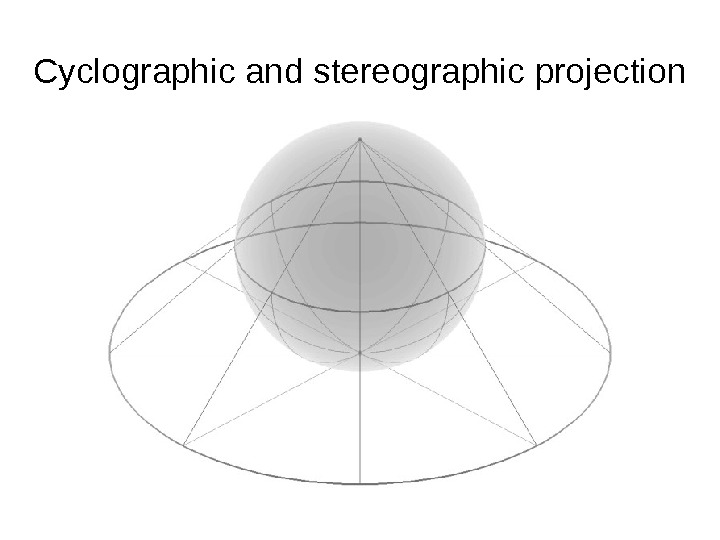Cyclographic and stereographic projection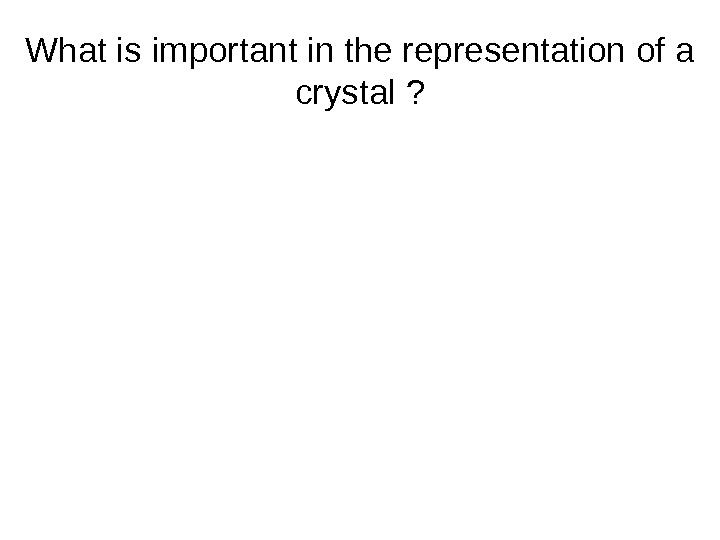What is important in the representation of a crystal ?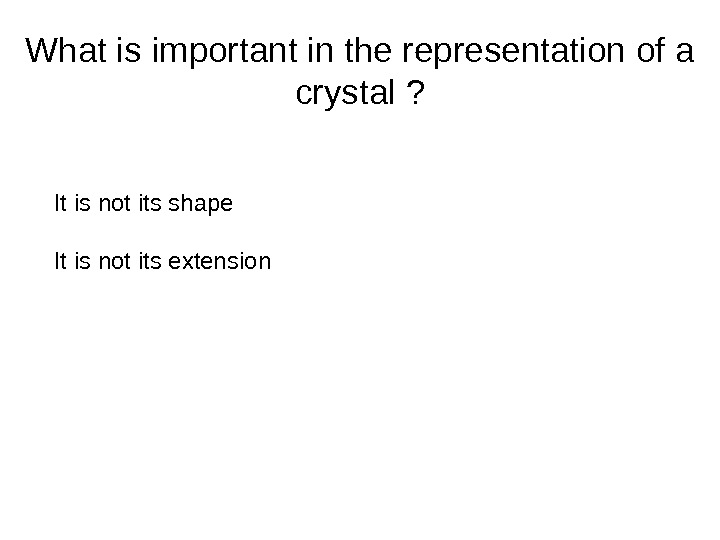What is important in the representation of a crystal ? It is not its shape It is not its extension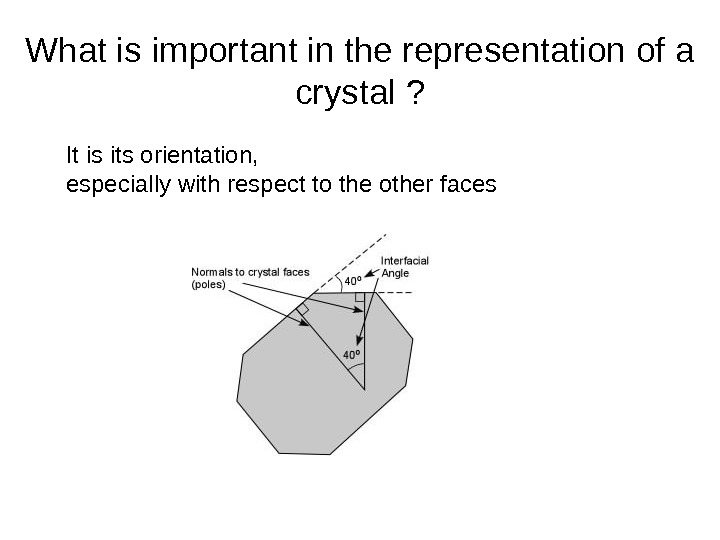What is important in the representation of a crystal ? It is its orientation, especially with respect to the other faces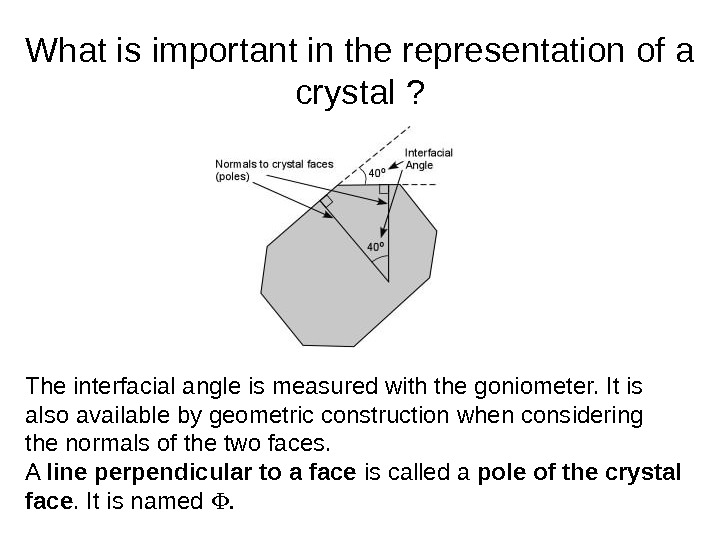What is important in the representation of a crystal ? The interfacial angle is measured with the goniometer. It is also available by geometric construction when considering the normals of the two faces. A line perpendicular to a face is called a pole of the crystal face. It is named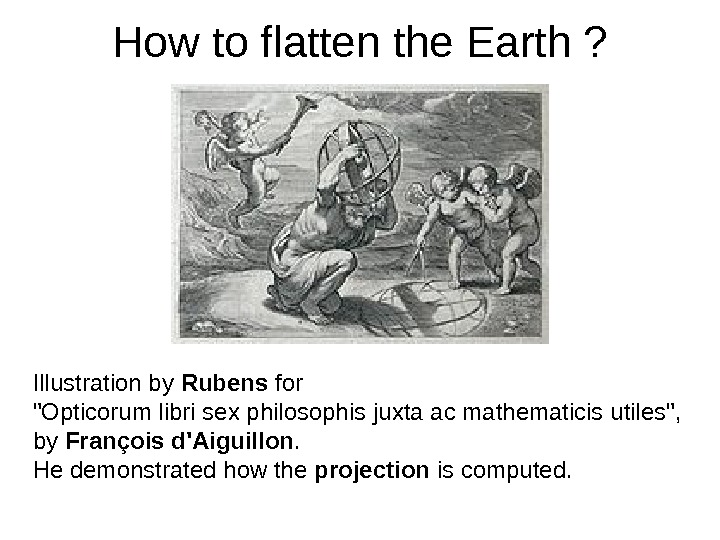How to flatten the Earth ? Illustration by Rubens for «Opticorum libri sex philosophis juxta ac mathematicis utiles», by François d’Aiguillon. He demonstrated how the projection is computed.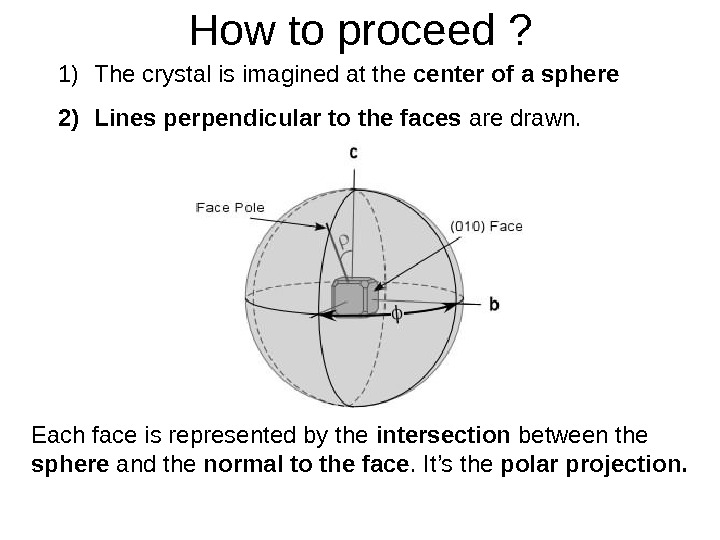How to proceed ? 1) The crystal is imagined at the center of a sphere 2) Lines perpendicular to the faces are drawn. Each face is represented by the intersection between the sphere and the normal to the face. It’s the polar projection.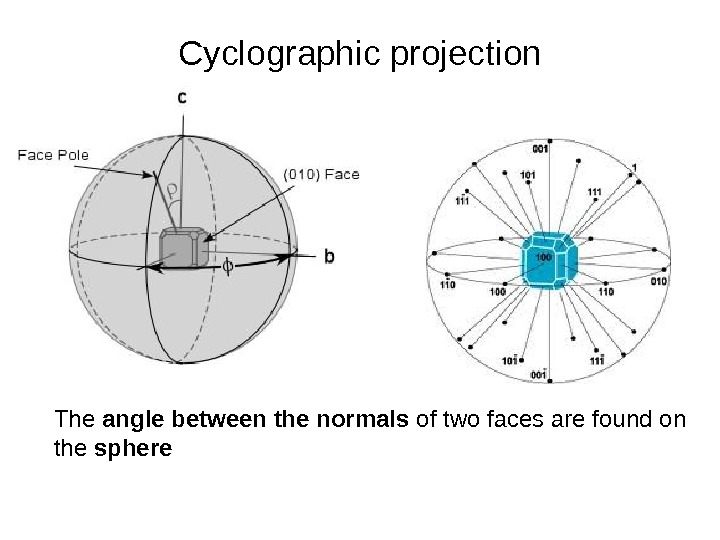Cyclographic projection The angle between the normals of two faces are found on the sphere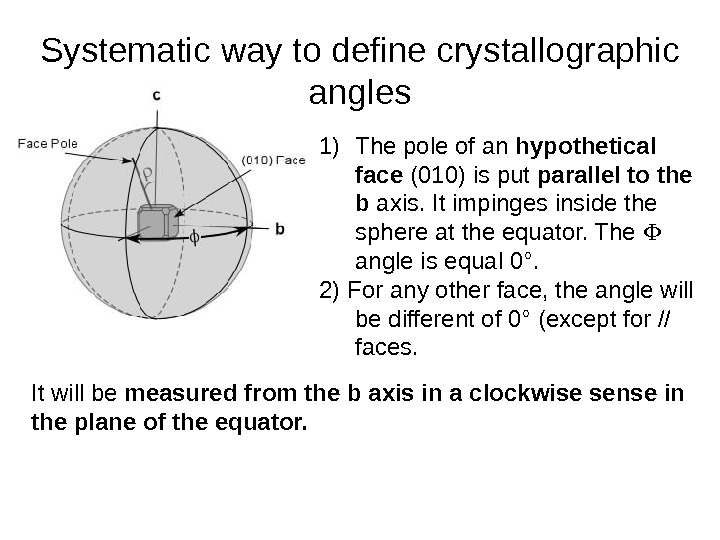Systematic way to define crystallographic angles 1) The pole of an hypothetical face (010) is put parallel to the b axis. It impinges inside the sphere at the equator. The angle is equal 0°. 2) For any other face, the angle will be different of 0° (except for // faces. It will be measured from the b axis in a clockwise sense in the plane of the equator.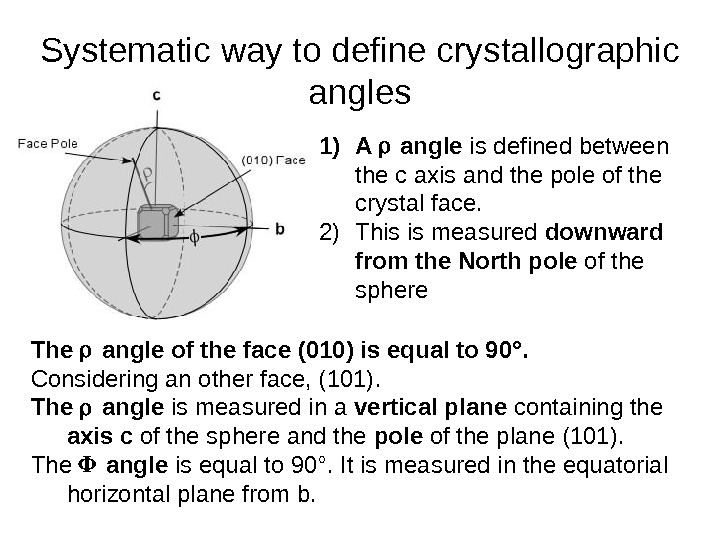Systematic way to define crystallographic angles 1) A angle is defined between the c axis and the pole of the crystal face. 2) This is measured downward from the North pole of the sphere The angle of the face (010) is equal to 90°. Considering an other face, (101). The angle is measured in a vertical plane containing the axis c of the sphere and the pole of the plane (101). The angle is equal to 90°. It is measured in the equatorial horizontal plane from b.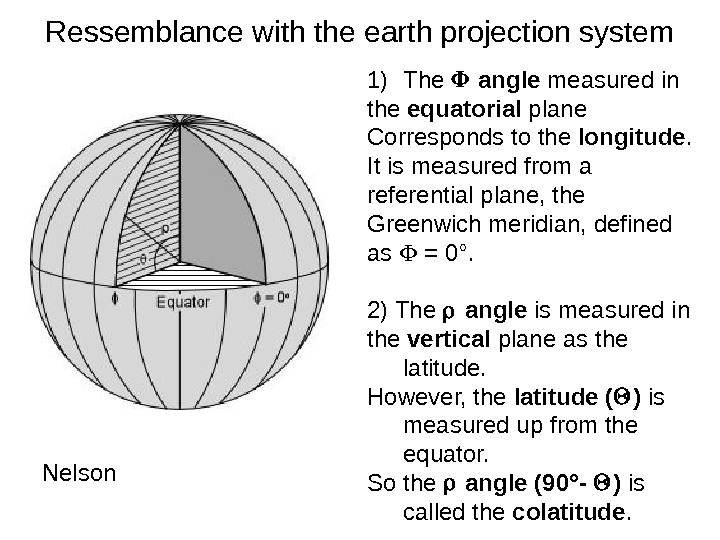Ressemblance with the earth projection system Nelson 1) The angle measured in the equatorial plane Corresponds to the longitude. It is measured from a referential plane, the Greenwich meridian, defined as = 0°. 2) The angle is measured in the vertical plane as the latitude. However, the latitude ( ) is measured up from the equator. So the angle (90°- ) is called the colatitude.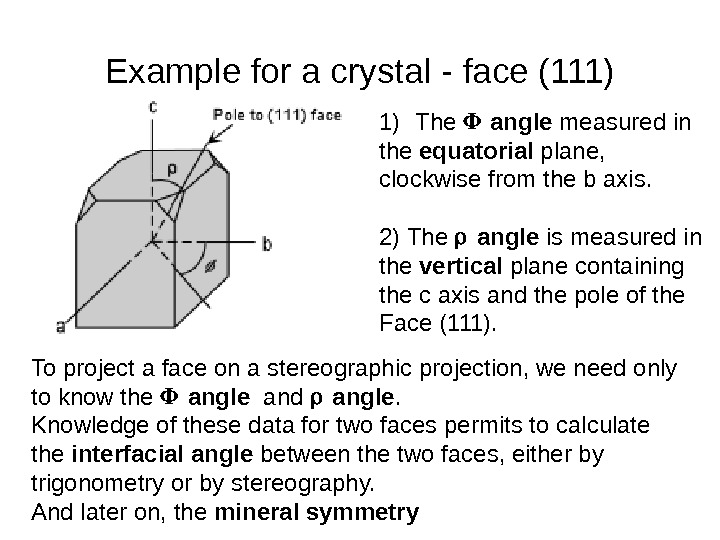Example for a crystal — face (111) 1) The angle measured in the equatorial plane, clockwise from the b axis. 2) The angle is measured in the vertical plane containing the c axis and the pole of the Face (111). To project a face on a stereographic projection, we need only to know the angle and angle. Knowledge of these data for two faces permits to calculate the interfacial angle between the two faces, either by trigonometry or by stereography. And later on, the mineral symmetry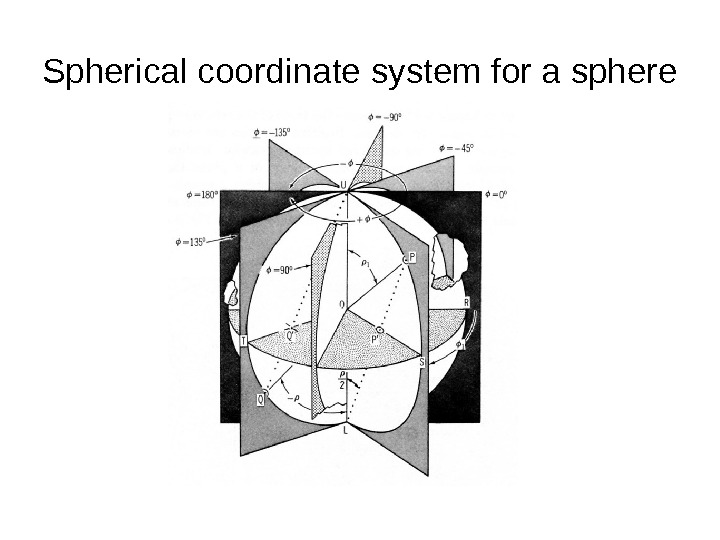Spherical coordinate system for a sphere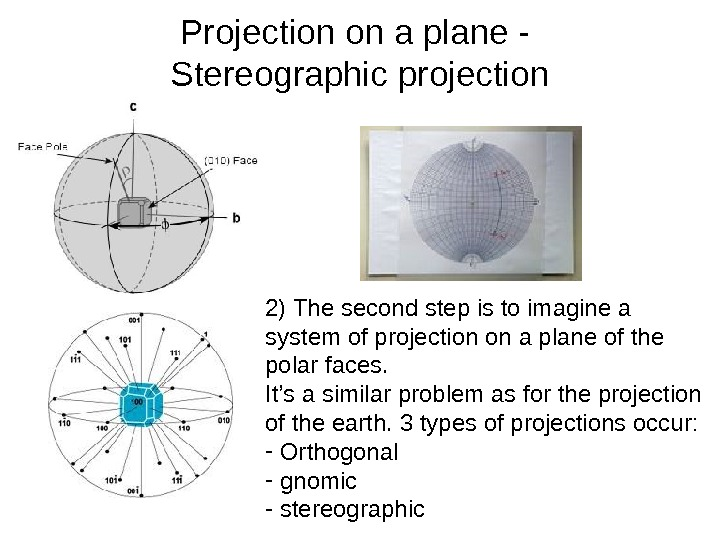Projection on a plane — S tereographic projection 2) The second step is to imagine a system of projection on a plane of the polar faces. It’s a similar problem as for the projection of the earth. 3 types of projections occur: — Orthogonal — gnomic — stereographic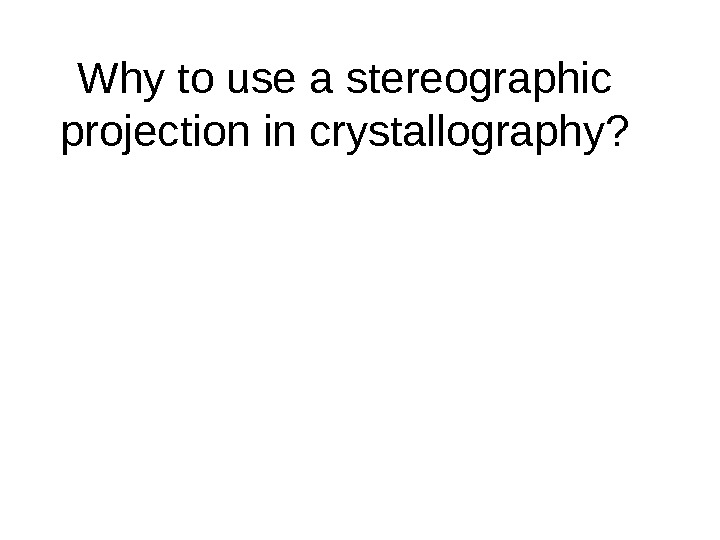Why to use a stereographic projection in crystallography?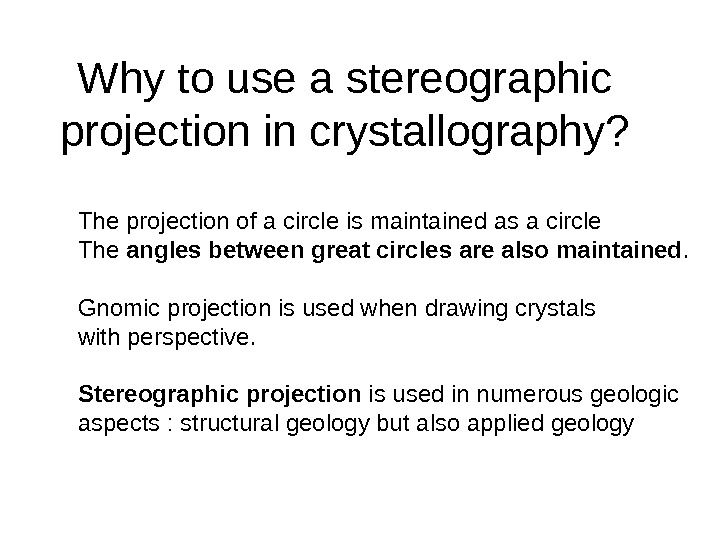Why to use a stereographic projection in crystallography? The projection of a circle is maintained as a circle The angles between great circles are also maintained. Gnomic projection is used when drawing crystals with perspective. Stereographic projection is used in numerous geologic aspects : structural geology but also applied geology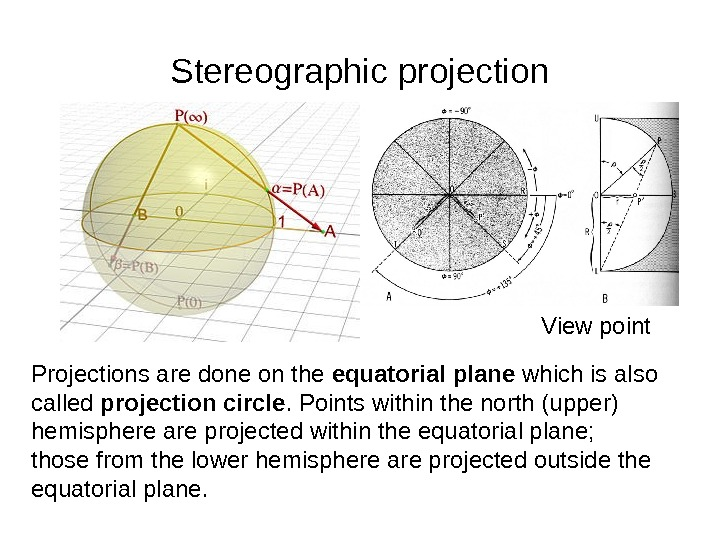Stereographic projection Projections are done on the equatorial plane which is also called projection circle. Points within the north (upper) hemisphere are projected within the equatorial plane; those from the lower hemisphere are projected outside the equatorial plane. View point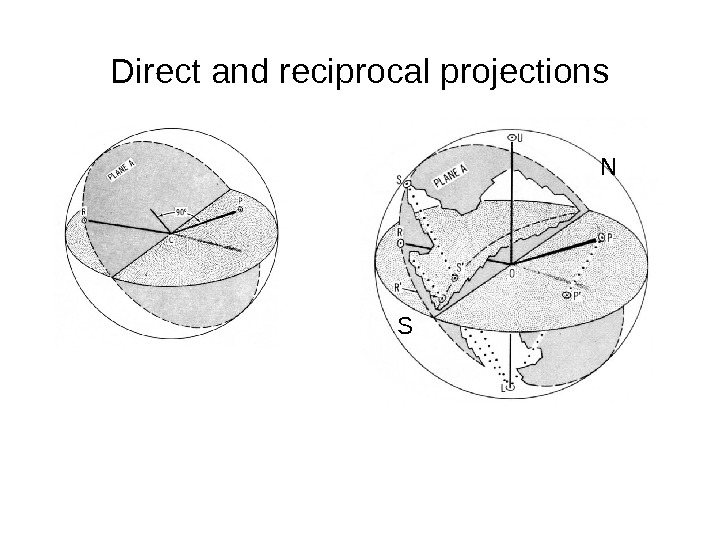Direct and reciprocal projections N S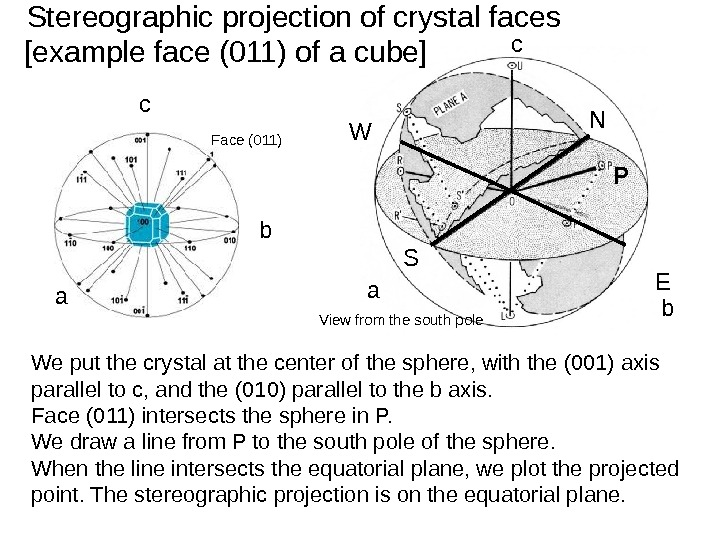Stereographic projection of crystal faces P P’ N S EW c ba View from the south polebc a Face (011)[example face (011) of a cube] We put the crystal at the center of the sphere, with the (001) axis parallel to c, and the (010) parallel to the b axis. Face (011) intersects the sphere in P. We draw a line from P to the south pole of the sphere. When the line intersects the equatorial plane, we plot the projected point. The stereographic projection is on the equatorial plane. P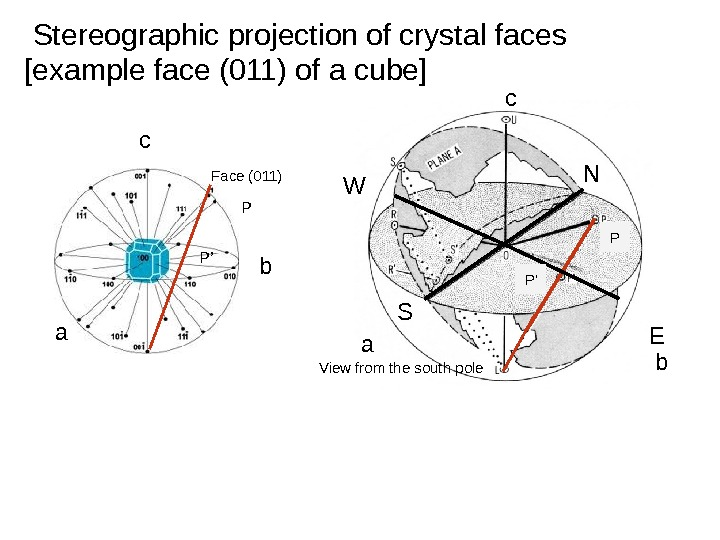Stereographic projection of crystal faces N S EW c ba View from the south pole P P’[example face (011) of a cube] bc a Face (011) P P’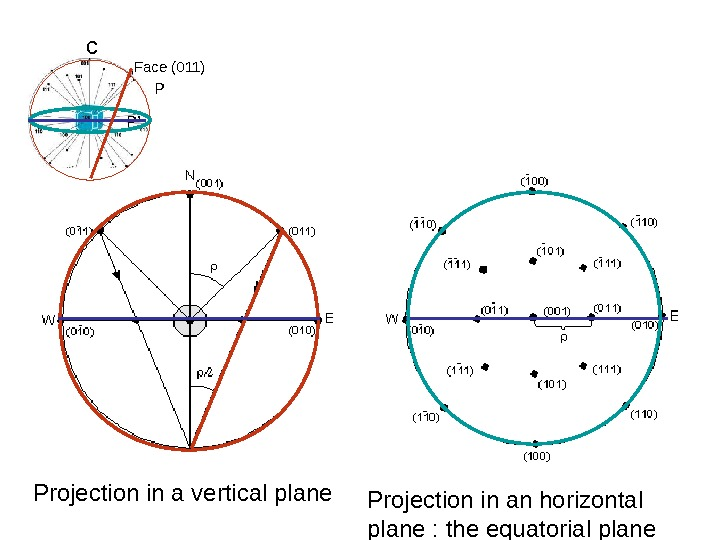c Face (011) P P’ Projection in a vertical plane Projection in an horizontal plane : the equatorial plane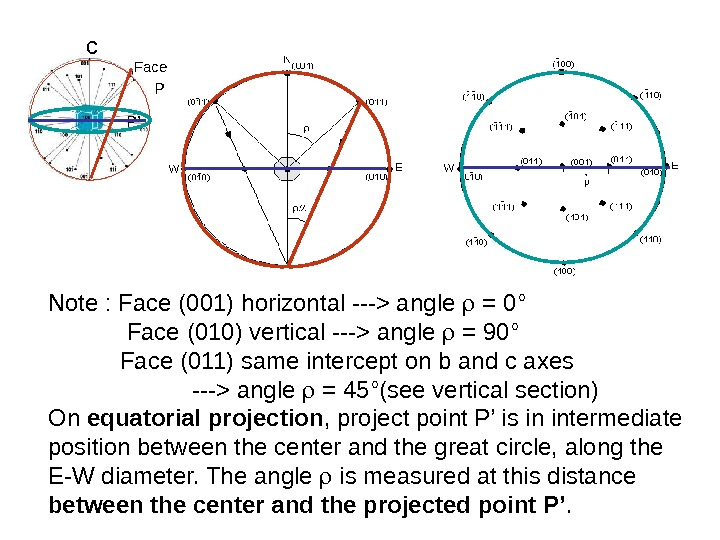c Face (011) P P’ Note : Face (001) horizontal —> angle = 0° Face (010) vertical —> angle = 90° Face (011) same intercept on b and c axes —> angle = 45°(see vertical section) On equatorial projection , project point P’ is in intermediate position between the center and the great circle, along the E-W diameter. The angle is measured at this distance between the center and the projected point P’.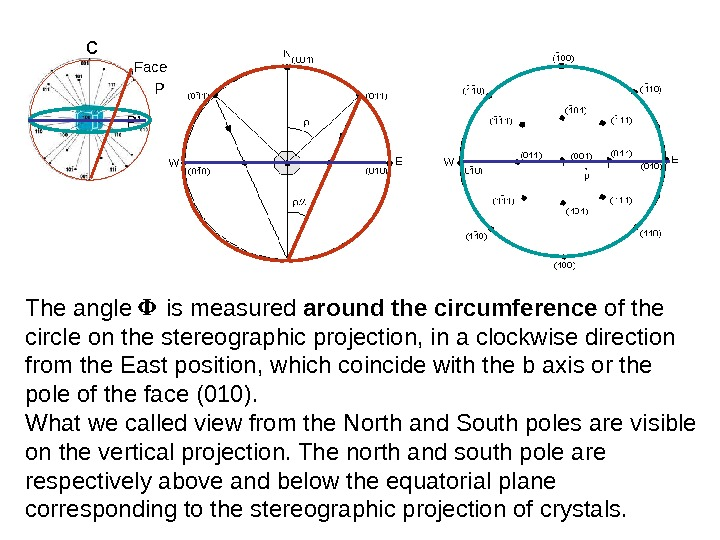c Face (011) P P’ The angle is measured around the circumference of the circle on the stereographic projection, in a clockwise direction from the East position, which coincide with the b axis or the pole of the face (010). What we called view from the North and South poles are visible on the vertical projection. The north and south pole are respectively above and below the equatorial plane corresponding to the stereographic projection of crystals.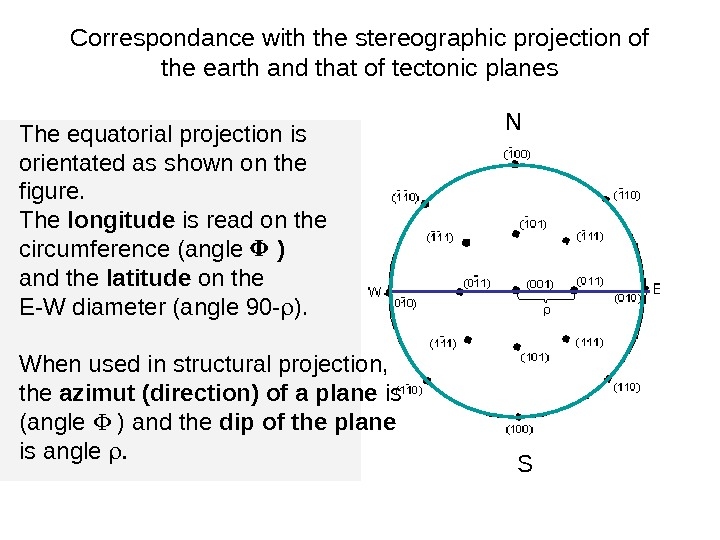Correspondance with the stereographic projection of the earth and that of tectonic planes N SThe equatorial projection is orientated as shown on the figure. The longitude is read on the circumference (angle ) and the latitude on the E-W diameter (angle 90 — ). When used in structural projection, the azimut (direction) of a plane is (angle ) and the dip of the plane is angle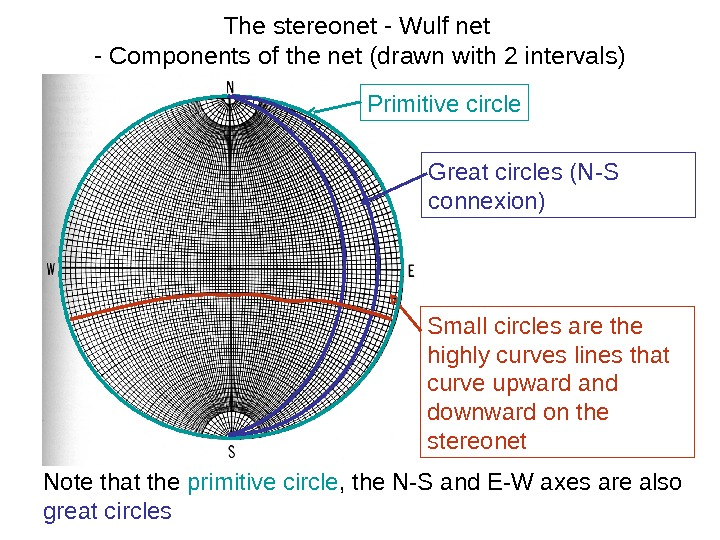The stereonet — Wulf net — Components of the net (drawn with 2 intervals) Primitive circle Great circles (N-S connexion) Small circles are the highly curves lines that curve upward and downward on the stereonet Note that the primitive circle , the N-S and E-W axes are also great circles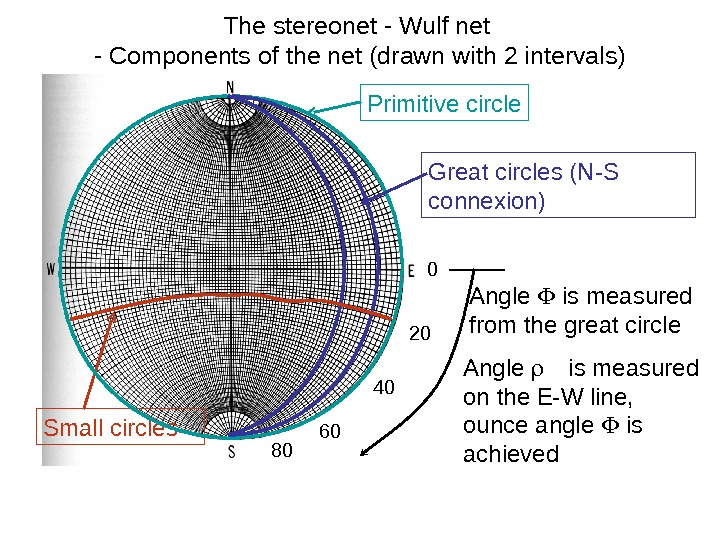The stereonet — Wulf net — Components of the net (drawn with 2 intervals) Primitive circle Great circles (N-S connexion) Small circles Angle is measured from the great circle 0 20 40 60 80 Angle is measured on the E-W line, ounce angle is achieved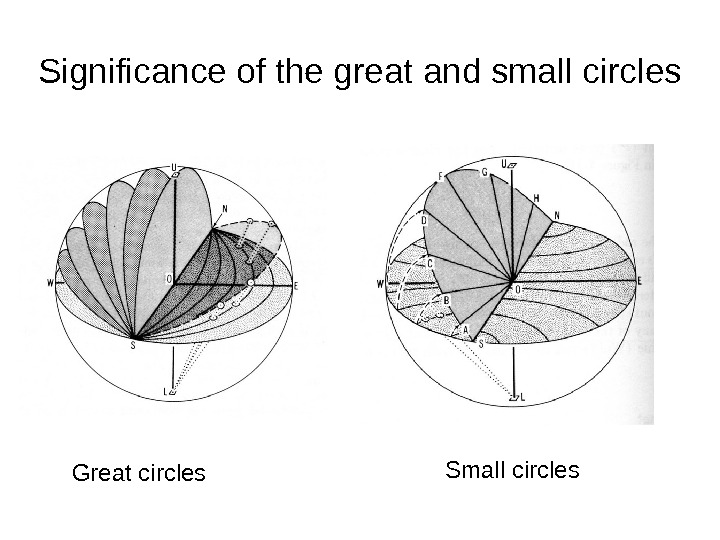Significance of the great and small circles Great circles Small circles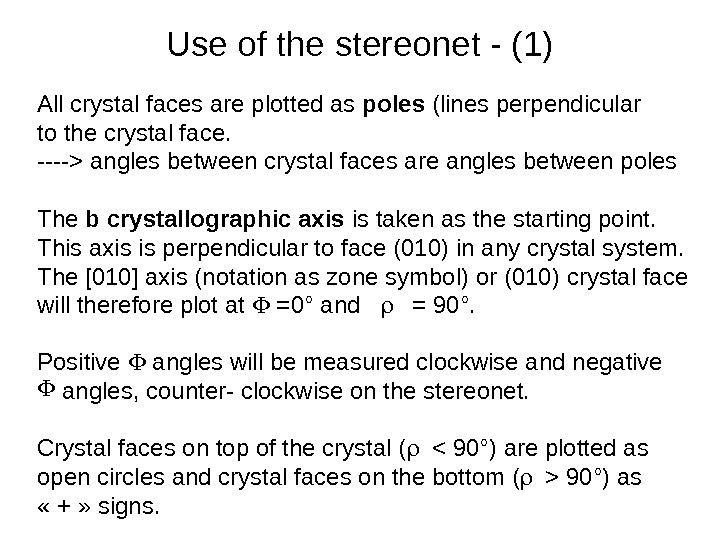Use of the stereonet — (1) All crystal faces are plotted as poles (lines perpendicular to the crystal face. —-> angles between crystal faces are angles between poles The b crystallographic axis is taken as the starting point. This axis is perpendicular to face (010) in any crystal system. The  axis (notation as zone symbol) or (010) crystal face will therefore plot at =0° and = 90°. Positive angles will be measured clockwise and negative angles, counter- clockwise on the stereonet. Crystal faces on top of the crystal ( 90°) as « + » signs.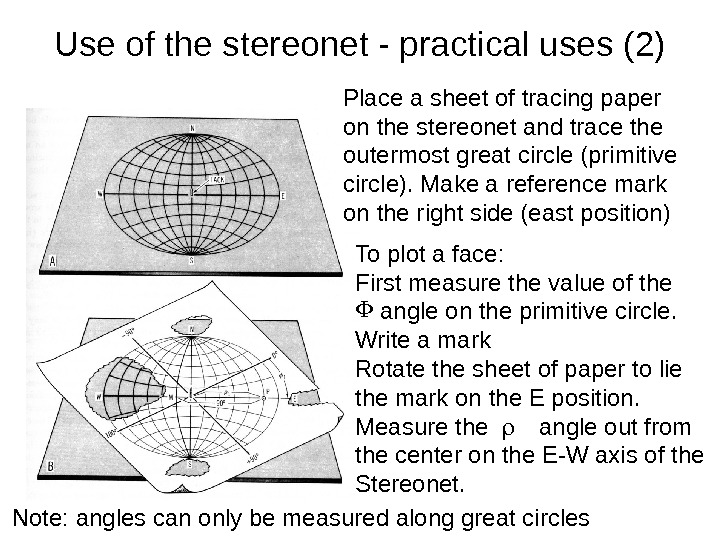Use of the stereonet — practical uses (2) To plot a face: First measure the value of the angle on the primitive circle. Write a mark Rotate the sheet of paper to lie the mark on the E position. Measure the angle out from the center on the E-W axis of the Stereonet. Place a sheet of tracing paper on the stereonet and trace the outermost great circle (primitive circle). Make a reference mark on the right side (east position) Note: angles can only be measured along great circles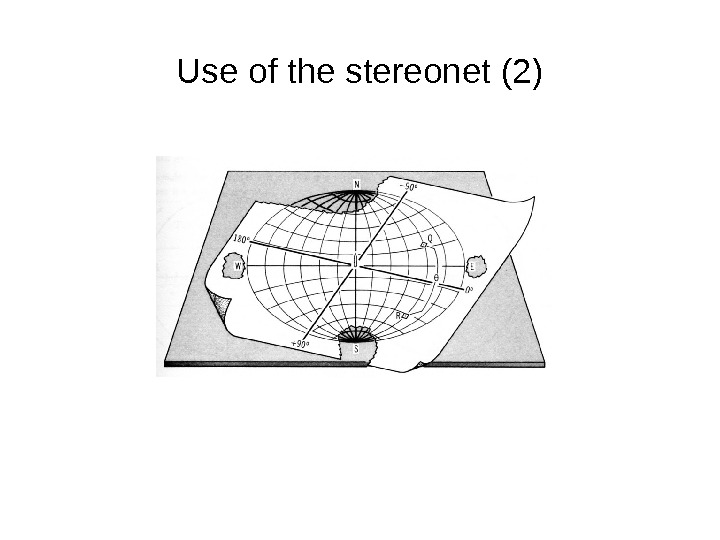Use of the stereonet (2)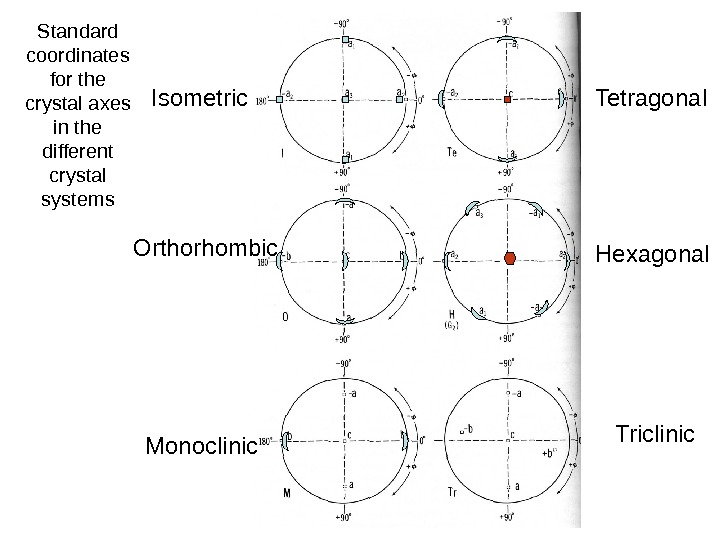Standard coordinates for the crystal axes in the different crystal systems Isometric Tetragonal Triclinic. Hexagonal. Orthorhombic Monoclinic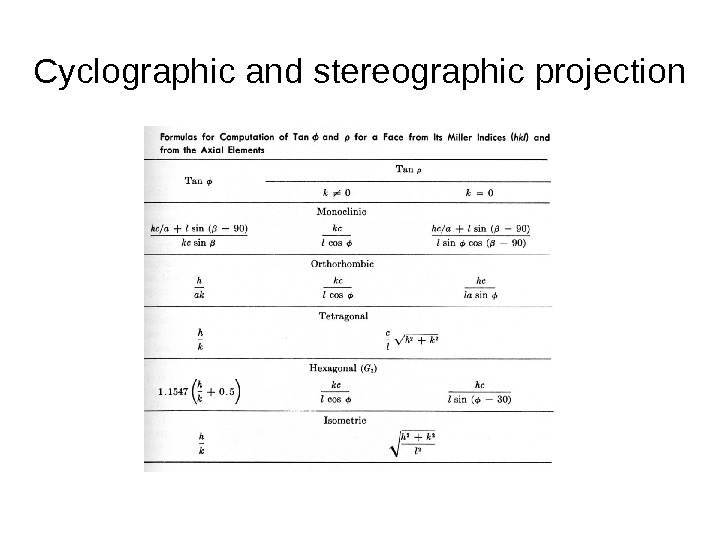Cyclographic and stereographic projection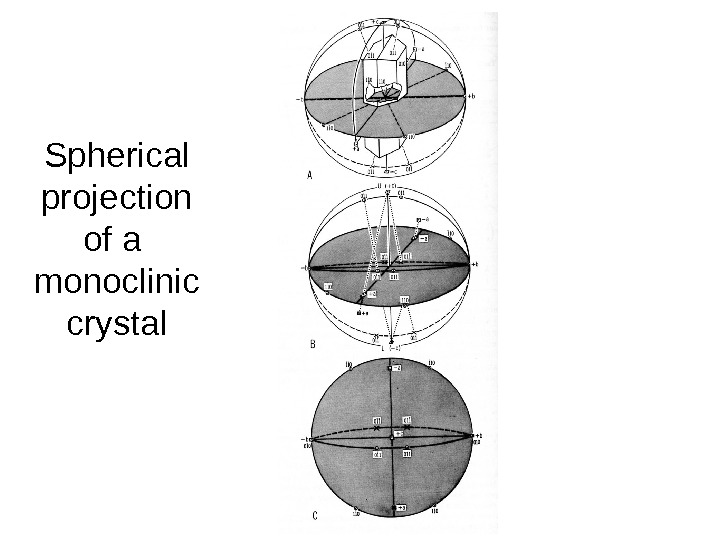Spherical projection of a monoclinic crystal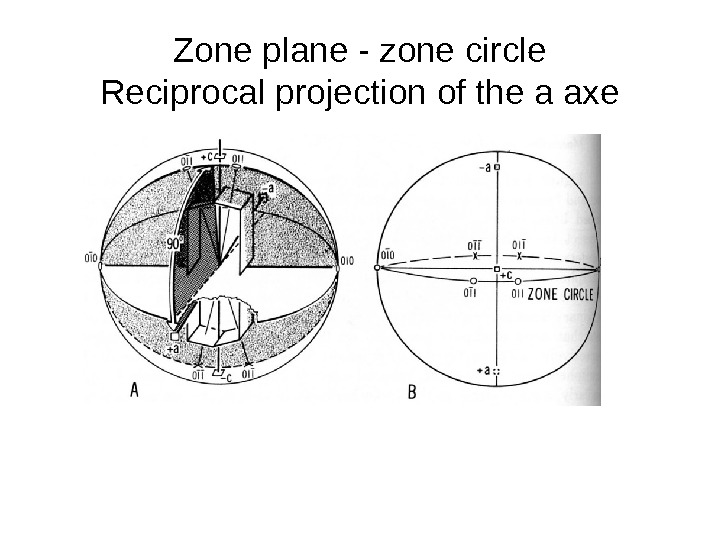Zone plane — zone circle Reciprocal projection of the a axe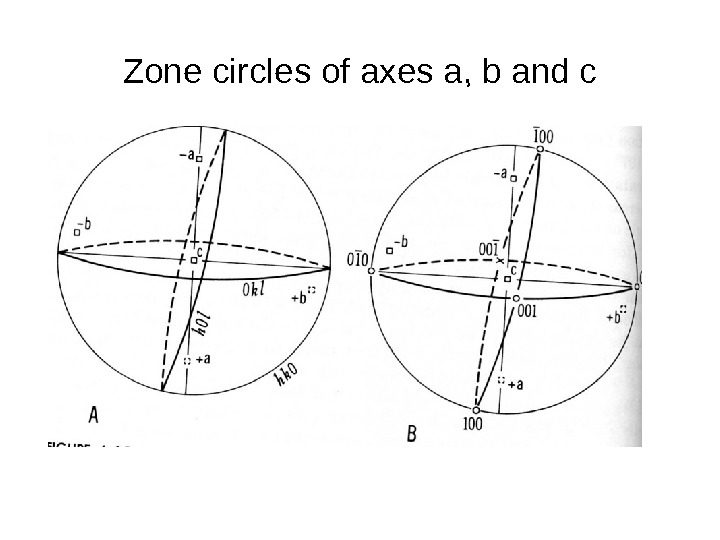Zone circles of axes a, b and c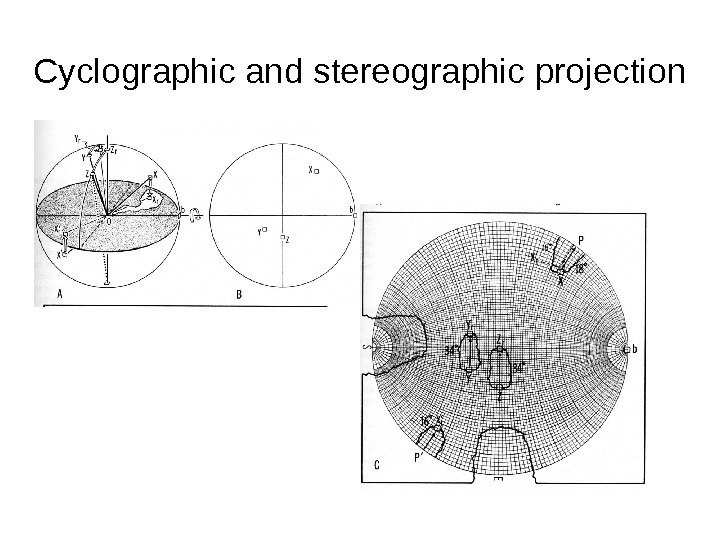Cyclographic and stereographic projectionCyclographic and stereographic projection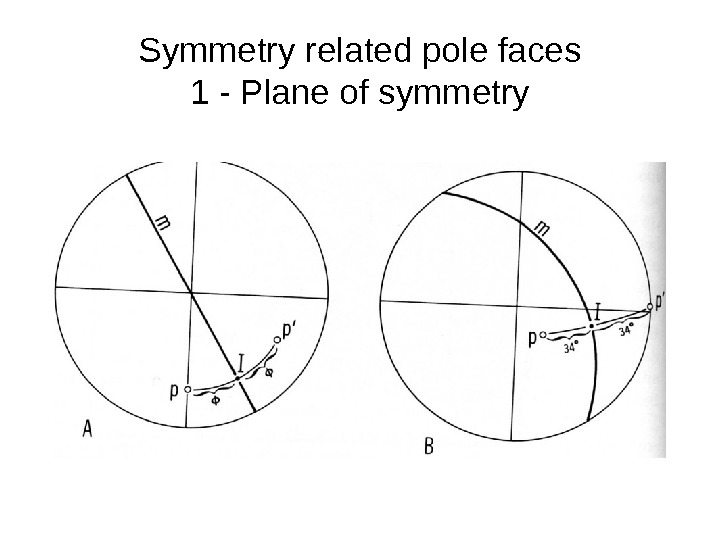Symmetry related pole faces 1 — Plane of symmetry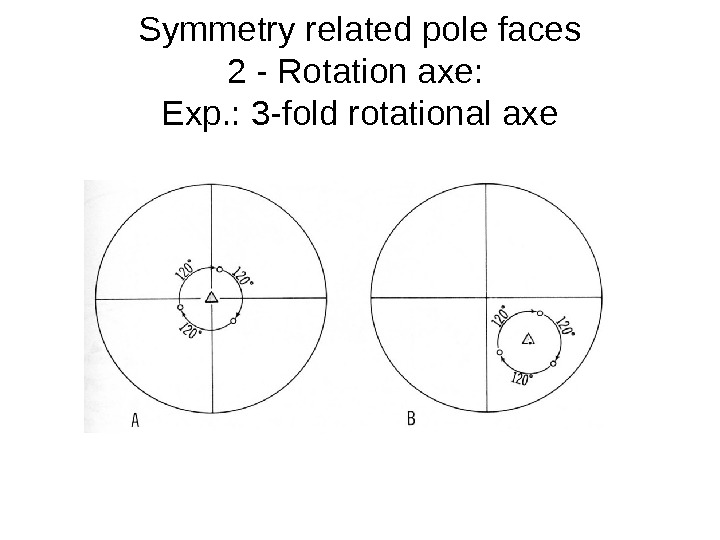Symmetry related pole faces 2 — Rotation axe: Exp. : 3 -fold rotational axe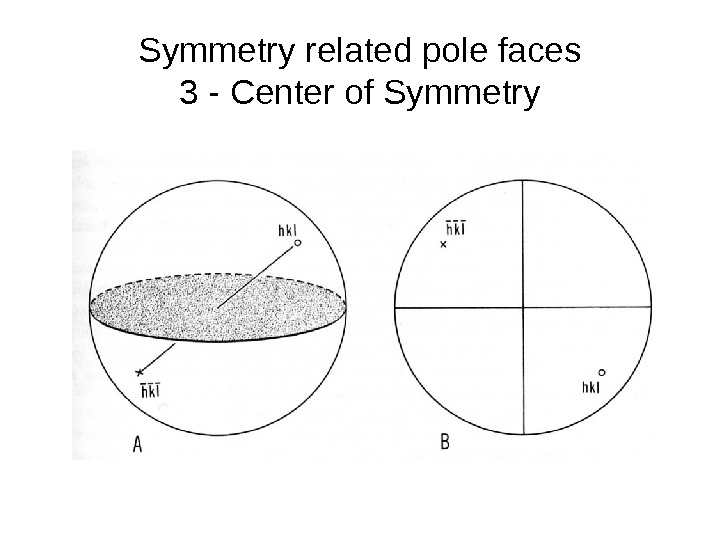Symmetry related pole faces 3 — Center of Symmetry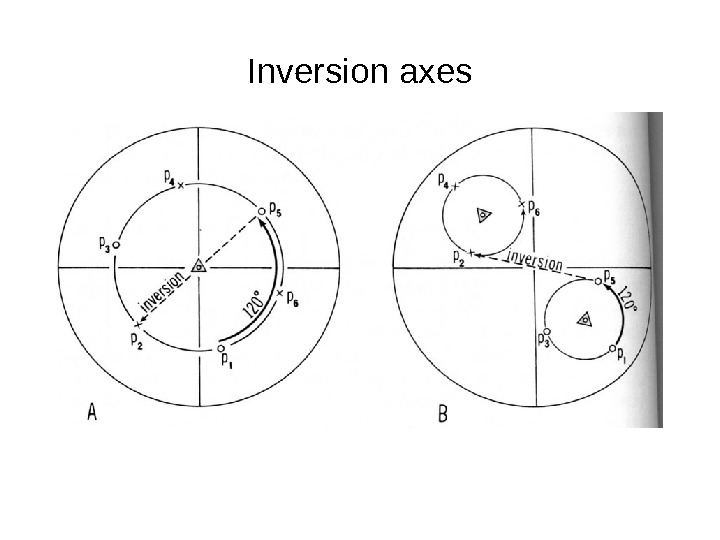Inversion axes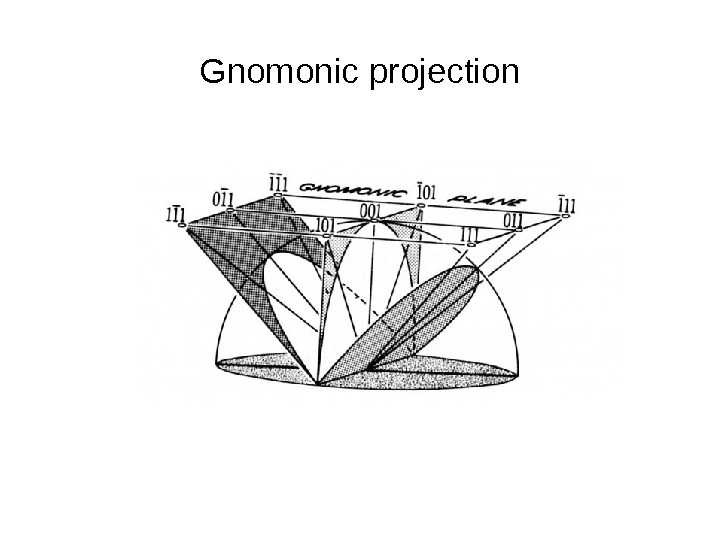Gnomonic projectionCyclographic and stereographic projection